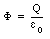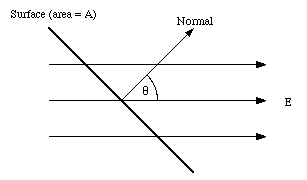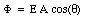#### Thank you for registering.

One of our academic counsellors will contact you within 1 working day.

Click to Chat

1800-5470-145

+91 7353221155

CART 0

• 0
MY CART (5)

Use Coupon: CART20 and get 20% off on all online Study Material

ITEM
DETAILS
MRP
DISCOUNT
FINAL PRICE
Total Price: Rs.

There are no items in this cart.
Continue Shopping

# explain gausss law?10 years ago

An alternative method to calculate the electric field of a given charge distribution relies on a theorem called Gauss' law. Gauss' law states that

" If the volume within an arbitrary closed mathematical surface holds a net electric charge Q, then the electric flux [Phi] though its surface is Q/[epsilon]0 "

Gauss' law can be written in the following form:(24.1)Figure 24.1. Electric flux through surface area A.

The electric flux [Phi] through a surface is defined as the product of the area A and the magnitude of the normal component of the electric field E:where [theta] is the angle between the electric field and the normal of the surface (see Figure 24.1). To apply Gauss' law one has to obtain the flux through a closed surface. This flux can be obtained by integrating eq.(24.2) over all the area of the surface. The convention used to define the flux as positive or negative is that the angle [theta] is measured with respect to the perpendicular erected on the outside of the closed surface: field lines leaving the volume make a positive contribution, and field lines entering the volume make a negative contribution.

plz approve if it is helpful...

10 years ago

Gauss's law states that:

The electric flux through any closed surface is proportional to the enclosed electric charge.

The law was formulated by Carl Friedrich Gauss in 1835, but was not published until 1867. It is one of four of Maxwell's equations which form the basis of classical electrodynamics, the other three being Gauss's law for magnetismFaraday's law of induction, and Ampère's law with Maxwell's correction. Gauss's law can be used to derive Coulomb's law, and vice versa.

Gauss's law may be expressed in its integral form:$\oint_S \mathbf{E} \cdot \mathrm{d}\mathbf{A} = \frac{Q}{\varepsilon_0},$

where the left-hand side of the equation is a surface integral denoting the electric flux through a closed surface S, and the right-hand side of the equation is the total charge enclosed by S divided by the electric constant.

Gauss's law also has a differential form:$\nabla \cdot \mathbf{E} = \frac{\rho}{\varepsilon_0}$

where  · E is the divergence of the electric field, and ρ is the charge density.

Good Luck!!!!!!!!!!!!!

Plz. approve by clicking 'Yes' given below!!!!!!!  Plz. Plz. Plz.!!!!!!!!1